MENU

Part number
Product ImagePackage
Dimensions
(mm)
Carrier Frequency
(kHz)
Transmission Distance Typ.
(m)
Detection Threshold / Sensitivity Typ.
Half Viewing Angle
(°)
Supply Current Typ.
(mA)
Pinning
Application
Mounting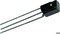Enlarge
Minicast 5.0 W x 6.95 H x 4.8 D 20 to 60 1.8 12 ± 45 0.7 1 = carrier OUT, 2 = GND, 3 = VS Code learning Leaded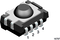Enlarge
Panhead 7.5 W x 5.3 H x 4.0 D 20 to 60 1.8 12 ± 50 0.7 1 = GND, 2 = N.C., 3 = VS, 4 = OUT Code learning SMD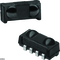Enlarge
Heimdall 6.8 W x 3.0 H x 3.2 D 20 to 60 1.8 12 ± 50 0.7 1, 4 = GND, 2 = VS, 3 = carrier OUT Code learning SMD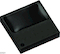Enlarge
Belobog 3.95 W x 3.95 H x 0.8 D 20 to 60 3 4 ± 75 0.37 1 = OUT, 2, 3, 6, 7, 8 = GND, 4, 5 = VS Code learning SMD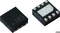Enlarge
VSOP 2.0 W x 2.0 H x 0.76 D 38 0.7 1, 4, 5 = N.C., 2 = VS, 3 = OUT, 6, 8 = GND, 7 = IN Code learning SMD